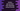# Python get quotient and remainder using divmod() method## Introduction :

Python divmod method can be used to find out the quotient and remainder at one go. It takes two numbers as arguments and returns the quotient and remainder as pair. For example, if the numbers are a and b, if both are integers, it will return results as a/b and a%b. For floating-point numbers, it will return math.floor(a/b)

### Example program :

Let’s take a look at the below program :

``````ans_1 = divmod(10,4)
print(ans_1)
print('quotient : {}, remainder : {}'.format(ans_1,ans_1))

ans_2 = divmod(100,13)
print(ans_2)
print('quotient : {}, remainder : {}'.format(ans_2,ans_2))``````

It will print the below output :

``````(2, 2)
quotient : 2, remainder : 2
(7, 9)
quotient : 7, remainder : 9``````